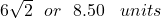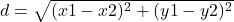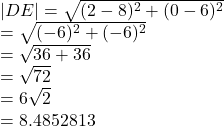## Use the Distance Formula to find the distance between D(2, 0) and E(8, 6).

Question

Use the Distance Formula to find the distance between D(2, 0) and E(8, 6).

in progress 0
3 months 2021-08-01T13:47:24+00:00 1 Answers 4 views 0

##Step-by-step explanation:

The distance between two points can be found by using the formulawhere

(x1 , y1) and (x2 , y2) are the points

From the question

The points are D(2, 0) and E(8, 6

The distance between them isWe have the final answer asHope this helps you